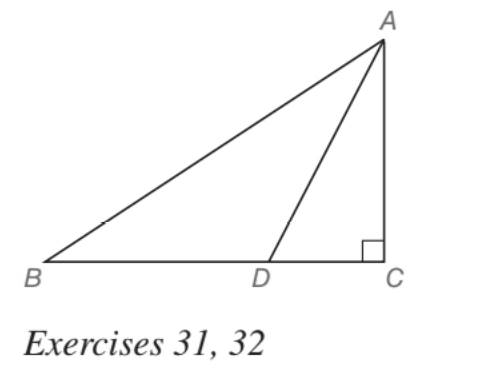Chapter 5.5, Problem 31EElementary Geometry For College St...

7th Edition
Alexander + 2 others
ISBN: 9781337614085

Solutions

Chapter
SectionElementary Geometry For College St...

7th Edition
Alexander + 2 others
ISBN: 9781337614085
Textbook Problem

In Exercises 27 to 33, give both exact solutions and approximate solutions to two decimal places. Given: Right ∆ A B C with m ∠ C = 90 ° and m ∠ B A C = 60 ° ; point D on B C ¯ ; A D ⃑ bisects ∠ B A C and A B = 12 Find: B DTo determine

To find:

BD in a right ABC such that mC=90° and mBAC=60°; point D on BC¯;AD

Bisects BAC and AB=12

Explanation

Approach:

For a right triangle, for which the measure of the interior angles 30°, 60°, and 90°; if ‘a’ is the length of measure of the shorter leg; opposite to the angle 30°, then the length of the other two sides is given by

Length of the longer leg (opposite to 60°) =a3

Length of the hypotenuse (opposite to 90°)=2a.

In general

Length of the longer leg =3× (Length of the shorter leg)

Length of the hypotenuse =2× (Length of the shorter leg)

Calculation:

Given,

A right ABC

mC=90° and mBAC=60°; point D on BC¯;AD

Bisects BAC and AB=12.

Since, AD bisects A of the ABC, we have

=12(60°)

First consider the right ABC to find AC and BC.

The above should be of the type 30°-60°-90° as such mA=60°, mC=90°.

Thus,

mB=30°

30°-60°-90° theorem.

In a right triangle whose angle measure 30°, 60°, and 90°, the hypotenuse has a length equal to twice the length of the shorter leg, and the longer leg is the product of 3 and the length of the shorter leg.

AB= Hypotenuse =12 units.

AC= Shorter leg, (opposite to 30°)

BC= Longer leg

Still sussing out bartleby?

Check out a sample textbook solution.

See a sample solution

The Solution to Your Study Problems

Bartleby provides explanations to thousands of textbook problems written by our experts, many with advanced degrees!

Get Started

Find the equilibrium point for the following supply and demand functions. Demand: Supp...

Mathematical Applications for the Management, Life, and Social Sciences

Write the sum in sigma notation. 19. x+x2+x3++xn

Single Variable Calculus: Early Transcendentals, Volume I

True or False: By the Integral Test, converges.

Study Guide for Stewart's Multivariable Calculus, 8th

True or False: If f(c) exists and f has a local minimum at c, then f(c) = 0.

Study Guide for Stewart's Single Variable Calculus: Early Transcendentals, 8th